# Elixir For Data Science# Digging For Golden Nuggets

Everyone wants to rest easy knowing that they are making the right decision because their decisions are based on data and facts. The problem is, there is too much data for any one person to consume manually, and the problem is getting worse every day. Despite this issue, your bosses, clients, and end users still need to make decisions going forward. How can you help them? This is where filtering comes in. Filtering is what helps you to dig through the mountain of data to find the "diamond in the rough" that can later become a shiny golden nugget.

Filtering is a data transformation method to remove unnecessary or unwanted rows of data. It prevents unnecessary data from affecting your model results later on, or when you start summarizing with counts, sums, and averages. For example, you may need to remove the `nil`s from your dataset for a model to run properly.

Without filtering properly, you may produce irrelevant or wrong answers due to “dirty“ data. In the same way, most people don’t want a pile of dirt. It doesn’t help them. They need your ability to go dig efficiently to find where the gold lies. Filtering is the preferred tool in your Data Transformation tool-bag to help you find the gems your clients depend on.

Below we'll cover the following sections:

## Starting Out

A good starting point would be to find all the flights from January 1st.

``````df
|> DataFrame.filter_with(
&(Series.equal(&1["month"], 1)
|> Series.and(Series.equal(&1["day"], 1)))
)
``````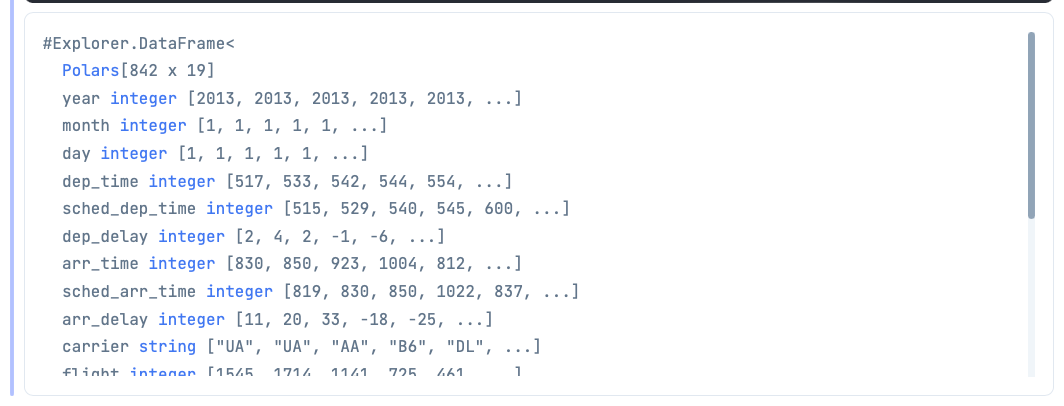Let's break down the 3 key functions that are used above:

1. `filter_with` -> Filters the rows of the DataFrame based on columns and conditions you provide.

2. `equal` -> Searches for the value (`1` and `1`) in the columns (`month` and `day`) and return where the instances are true.

3. `and` -> Combines both `equal` searches to find all the instances where both the `month` column == 1 and the `day` column == 1.

## Comparisons

Comparisons are what you use to determine whether two items are equivalent or not. If so, the comparison will return `true`, and if not, it will return `false`.

Explorer handles equality for you with the `equal` function (as demonstrated above), so you don't need to worry about using `=` vs `==` vs `===` like you normally would.

For now, refer to the simple example below for a key point to be aware of when making comparisons.

``````:math.sqrt(2) |> :math.pow(2) == 2

# false
``````

Notice how the result is `false`. ButThe square root of 2 raised to the 2nd power should equal 2. Why is that? Looking at the first two operations will give you a hint.

``````:math.sqrt(2) |> :math.pow(2)

# 2.0000000000000004
``````

Although the answer should be 2, you can see that it isn't. This is due to computers having finite memory and needing to limit the number of decimals they can store. Thus, there is some error introduced due to rounding.

Notice how you have the same problem in the example below.

``````1 / 49 * 49 == 1

# false
``````

Elixir does not have a `near` function, like R does, to handle whether two numbers are approximately correct. One solution to this problem would be to use the `round` function.

``````# Take the square root
:math.sqrt(2)
# Raise it to the power of 2
|> :math.pow(2)
# Round the number to the nearest whole number
|> Float.round() == 2

# true
``````

The same is also true for the second problem

``````(1 / 49 * 49) |> Float.round() == 1

# true
``````

Notice how, using the `round` function, you were able to "undo" the effects of the rounding that the computer made when calculating the formula in the first place.

## Logical Operators

Logical operators can often be tricky and can get complex quickly when dealing with more than one. In the book R For Data Science, there is an excellent Venn diagram (shown below) to help you remember how and (&), or (|) and not (!) work together to create the right combination of two datasets.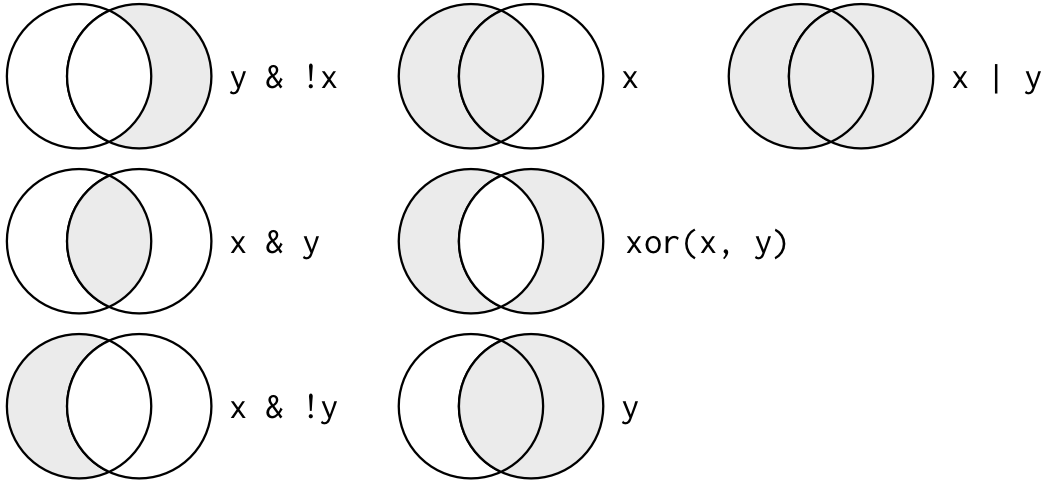‌To start, find all the flights that departed in November or December.

``````df
|> DataFrame.filter_with(
&(Series.equal(&1["month"], 11)
|> Series.or(Series.equal(&1["month"], 12)))
)
``````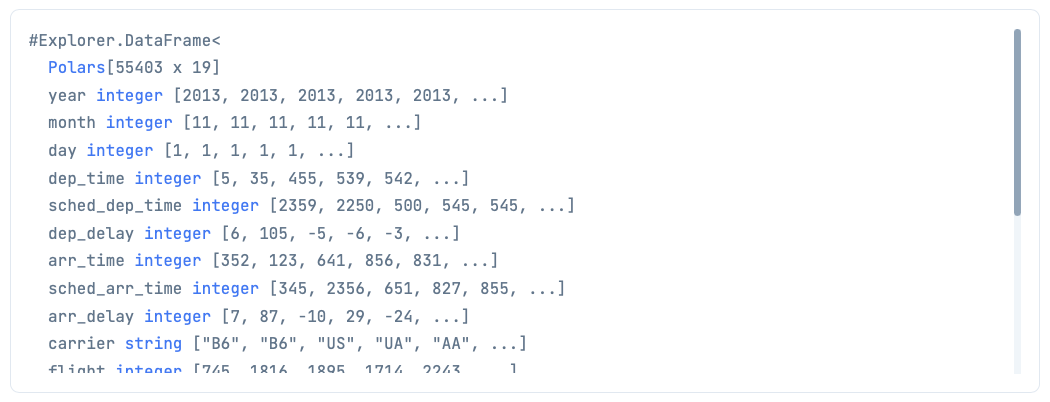…but what if you also wanted to include October?

You can create a pipe within a pipe by combining the `equal` and `or` functions within the `Series` module to solve this.

``````df
|> DataFrame.filter_with(
&(Series.equal(&1["month"], 11)
|> Series.or(Series.equal(&1["month"], 12))
|> Series.or(Series.equal(&1["month"], 10)))
)
``````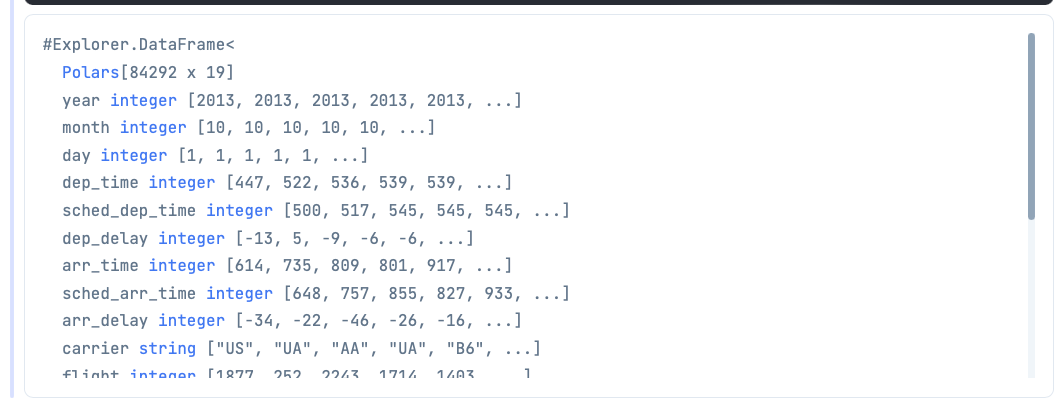Note: As of this writing, you cannot use the `*!*` to create the opposite of the filter you are creating. Simply use the opposite function like `*less_equal*` (<=) instead of `*greater*` (>). Or, you could use `*greater_equal*` (>=) instead of `*less*` (<).

Note: `*\|\|*` and `*&&*` will not work in place of `*Series.or*` and `*Series.and*` for combining conditions.

Next, try finding the flights that weren't delayed (arrival or departure) by more than 2 hours.

``````df
|> DataFrame.filter_with(
&(Series.less_equal(&1["arr_delay"], 120)
|> Series.or(Series.less_equal(&1["dep_delay"], 120)))
)
``````

Note: 120 minutes is used in place of 2 hours since the `arr_delay` and `dep_delay` are in minutes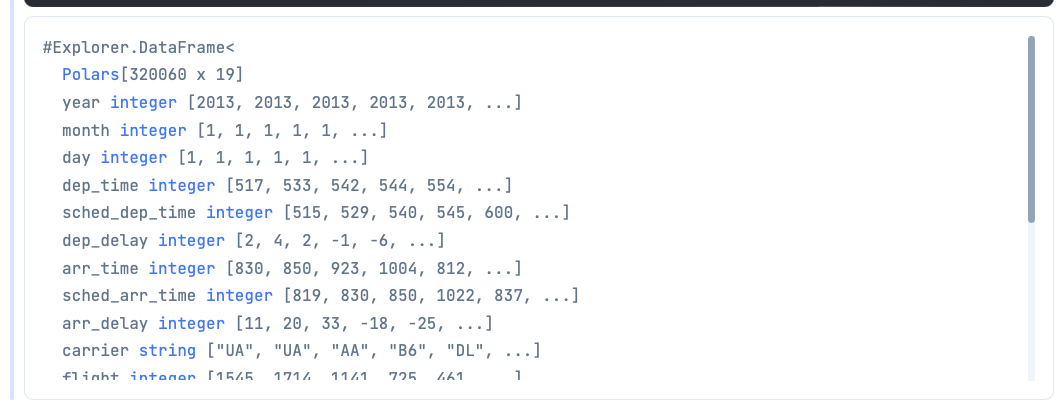# Missing Values

Missing values occur in almost every dataset. Thus, you need to have a way to handle and work with them. Below are a couple of functions that you can use with and without DataFrames.

`#N/A` in R is equivalent to `nil` in Elixir. The only difference is, in Elixir, you won't get an error when comparing `nil`s.

``````nil == nil
# true
``````

You can check for `nil` with `is_nil`.

``````x = nil

is_nil(x)

# true
``````

`Filter` in Explorer does not automatically remove `nil`s from the dataset. You must explicitly do so if you want them removed.

Note: You can drop all rows with `nil`s by using the `drop_nil` function.

``````df
|> DataFrame.drop_nil()
``````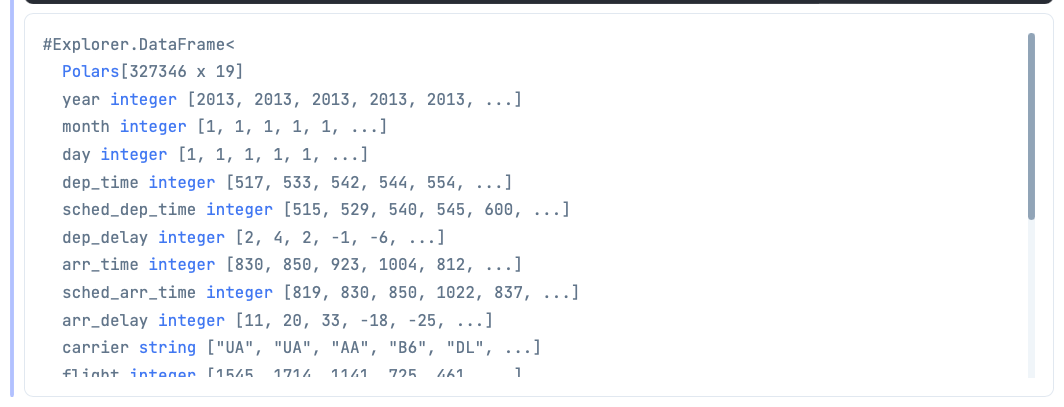Now, say you wanted to avoid removing every row with a `nil` from your DataFrame, but simply wanted to remove all the `nil`s from a specific column. How would you go about that? This is where the `is_nil` function comes in. It will find all the `nil` rows in a column, so you can either keep or remove them.

With that, find all the missing `air_time` data.

``````df
|> DataFrame.filter_with(&Series.is_nil(&1["air_time"]))
``````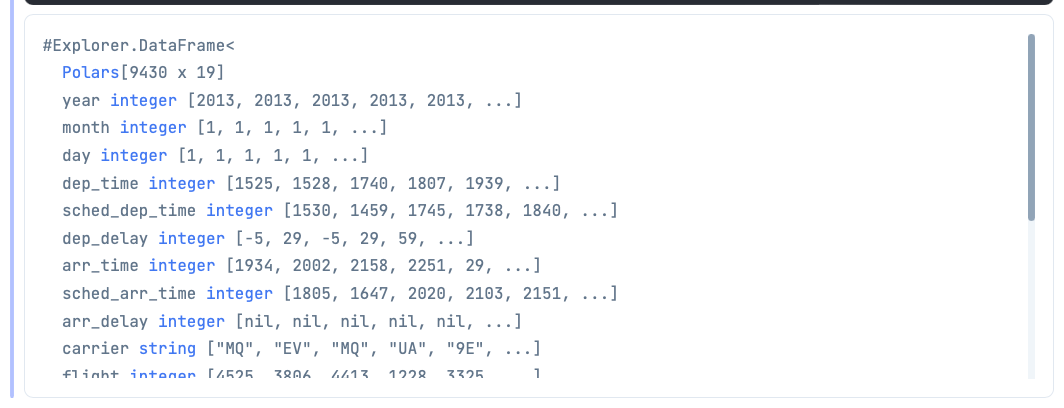‌Although you may have a feel for how filtering works in Explorer, you've just scratched the surface of what can be done with filtering from the examples above. If you'd like more examples, you can dig into the documentation.